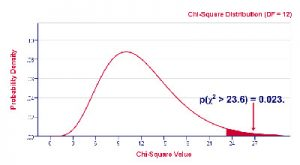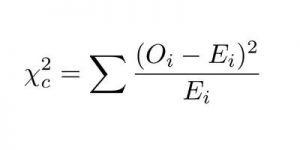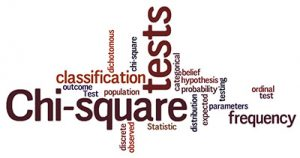# Chi Square Calculator

Use this Chi Square calculator to get a critical value for a Chi Squared distribution

Chi-Square Table Value Calculator

## What Is The Chi Square Test For?

The Chi square test should be run when you want to test how likely it is that an observed distribution is motivated by chance. This is also called a goodness of fit statistic since it measures how well the observed data actually fits with the distribution that you expect to see if the variables are independent. Make sure that you use the chi square formula to determine the chi square value.One of the things that you need to know about the chi square test is that it only analyzes categorical data. This means that you will need to count the data and divided it into categories. Notice that the chi square test can’t be run with continuous or paramedic data such as weight in pounds, for example. Make sure to confirm your results with our chi square calculator.

## The Chi Square Test – A Practical Example

Let’s say that you want to test whether attending classes has any influence on how students perform on an exam. Since exams usually use test scores between 0 and 100, you can’t use this data as it is with a chi square test since these are numerical variables. So, one of the things that you can do is to create two categories – “Pass” and “Fail”. Besides, since the chi square test doesn’t handle percentages either, by dividing a class of 54 into groups according to whether they attended class and whether they passed the exam, you might construct a data set like this:

 Pass Fail Attended 25 6 Skipped 8 15

One of the most important things that you need to pay attention to is on how you are building the different categories. The truth is that the chi square test will tell you the information depending on how you divide your data. So, you want to ensure that you divide it into a way that you can get meaningful categories. After collecting the data, you should use the chi square formula to determine the chi square.

Let’s say that you are working with data that has groups of people. If you think about it, you can divide them in many different ways. You can find categories for age groups, gender, income, dead or alive, among so many others. So, ultimately, you need to think about the main purpose of why you’re doing the chi square test and decide on the best categories.

## Defining The Chi Square Test In A Different Way

While you may have understood why the chi square test is performed, there may be other situations where you may be in doubt if you can use this test or not. So, we believe that a good approach is to show you as many examples as we can of different situations where you can use the chi square test. Nevertheless, you should always use the chi square formula.When you are looking at statistical language, it is frequent that a way to describe the chi square test is that it tests the null hypothesis that the variables are independent. So, simply put, the test will compare the observed data with a model that distribute the data according to the expectation that the variables are independent. Wondering about the results you got? Confirm them with our chi square calculator.

Let’s take a look at a practical example.

On the following table, we have the representation of the input you need to conduct a chi square test using two variables to divide the data: the gender and the party affiliation.

 Democrat Republican Male 20 30 Female 30 20

As you can easily see, the previous table has a 2 X 2 grid. However, it is incomplete. Why? The truth is that it should include the total counts for each column and row, as well as for the entire data set:

 Democrat Republican Total Male 20 30 50 Female 30 20 50 Total 50 50 100

So, now we have all the data set that we need. This data set includes 100 individuals that fit a specific gender (male or female) as well as their party affiliation (democrat or republican).

When you use a chi square test in this situation, you will be able to determine the likelihood of a gender and the party affiliation to be completely independent. If you prefer, you can also say that you are trying to determine how likely it is that the distribution of males and females in each party is due to chance.

As you can easily discover, the null hypothesis in this specific case will be that the gender and party affiliation are independent of one another. So, to ensure that you test this hypothesis, you will need to create a model that can estimate how the data is distributed if your hypothesis of independence is correct. And this is the reason why you need the totals on your tables. Discover the chi square value using our free chi square calculator.

Let’s assume that you have a 50/50 chance of females and males being in either party:

 Democrat Republican Total Male 25 25 50 Female 25 25 50 Total 50 50 100

Situations When You Shouldn’t Use The Chi Square Test

While you can use the chi square test in many different situations, there are some occasions when you shouldn’t use it.

One of the things that you need to keep in mind is that the chi square test should only be used to test the probability of independence of a distribution of data. However, it is important to clearly state that the chi square test won’t provide you any details about the relationship between these variables whatsoever. This means that if you were looking to know how likely it is for a woman to be a democrat than a man, the Chi square wouldn’t be the better test for this.Besides, when you are thinking of using the chi square test, you want to ensure that you organize your data the best way you can so that you can answer the question that you have. So, you want to ensure that the variables that you use are mutually exclusive which means that if it participates in one category, it can’t be in a different category. This means that you won’t be counting any item more than once.

As an additional consideration, you want to make sure that you never exclude a part of your data set. Let’s say that the data you collected for the previous example had also got some answers for Independent besides Democratic and Republican. You want to ensure that you add this category to the test.